×#### Thank you for registering.

One of our academic counsellors will contact you within 1 working day.

Click to Chat

1800-1023-196

+91-120-4616500

CART 0

• 0

MY CART (5)

Use Coupon: CART20 and get 20% off on all online Study Material

ITEM
DETAILS
MRP
DISCOUNT
FINAL PRICE
Total Price: Rs.

There are no items in this cart.
Continue Shopping• Complete JEE Main/Advanced Course and Test Series
• OFFERED PRICE: Rs. 15,900
• View Details

```Revision Notes on Rational Numbers

Natural Numbers

All positive integers like 1, 2, 3, 4……..are natural numbers.

Whole Numbers

All natural numbers including 0 are whole numbers.

Integers

All negative and positive numbers including 0 are called Integers.

Rational Numbers

Rational Numbers are the numbers that can be expressed in the form p/q where p and q are integers (q ≠ 0). It includes all natural, whole numbers, fractions and integers.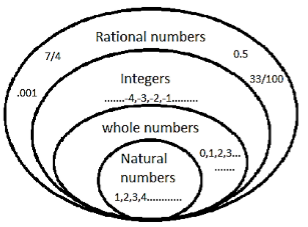Equivalent Rational Numbers

By multiplying or dividing the numerator and denominator of a rational number by the same integer, we can obtain another rational number equivalent to the given rational number.

Numbers are said to be equivalent if they are proportionate to each other.

Example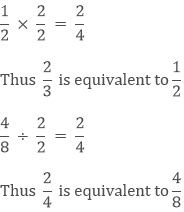Therefore 1/2, 2/4, 4/8 are equivalent to each other as they are equal to each other.

Positive and Negative Rational Numbers

1. Positive Rational Numbers are the numbers whose both the numerator and denominator are positive.

Example: 3/4, 12/24 etc.

2. Negative Rational Numbers are the numbers whose one of the numerator or denominator is negative.

Example: (-2)/6, 36/(-3) etc.

Remark: The number 0 is neither a positive nor a negative rational number.

Rational Numbers on the Number Line

Representation of whole numbers, natural numbers and integers on a number line is done as followsRational Numbers can also be represented on a number line like integers i.e. positive rational numbers are on the right to 0 and negative rational numbers are on the left of 0.

Representation of rational numbers can be done on a number line as follows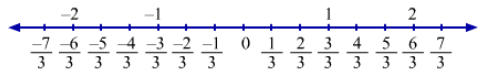Rational Numbers in Standard Form

A rational number is in the standard form if its denominator is a positive integer and there is no common factor between the numerator and denominator other than 1.

If any given rational number is not in the standard form then we can reduce it to its standard form or the lowest form by dividing its numerator and denominator by their HCF ignoring its negative sign.

Example

Find the standard form of 12/18

Solution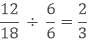2/3 is the standard or simplest form of 12/18

Comparison of Rational Numbers

1. To compare the two positive rational numbers we need to make their denominator same, then we can easily compare them.

Example

Compare 4/5 and 3/8 and tell which one is greater.

Solution

To make their denominator same, we need to take the LCM of the denominator of both the numbers.

LCM of 5 and 8 is 40.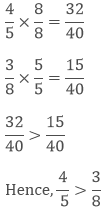2. To compare two negative rational numbers, we compare them ignoring their negative signs and then reverse the order.

Example

Compare - (2/5) and – (3/7) and tell which one is greater.

Solution

To compare, we need to compare them as normal numbers.

LCM of 5 and 7 is 35.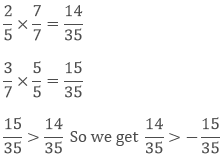by reversing the order of the numbers.3. If we have to compare one negative and one positive rational number then it is clear that the positive rational number will always be greater as the positive rational number is on the right to 0 and the negative rational numbers are on the left of 0.

Example

Compare 2/5 and – (2/5) and tell which one is greater.

Solution

It is simply that 2/5 > - (2/5)

Rational Numbers between Rational Numbers

To find the rational numbers between two rational numbers, we have to make their denominator same then we can find the rational numbers.

Example

Find the rational numbers between 3/5 and 3/7.

Solution

To find the rational numbers between 3/5 and 3/7, we have to make their denominator same.

LCM of 5 and 7 is 35.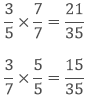Hence the rational numbers between 3/5 and 3/7 are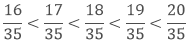These are not the only rational numbers between 3/5 and 3/7.

If we find the equivalent rational numbers of both 3/5 and 3/7 then we can find more rational numbers between them.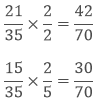Hence we can find more rational numbers between 3/5 and 3/7.

Remark: There are “n” numbers of rational numbers between any two rational numbers.

Operations on Rational Numbers

a. Addition of two rational numbers with the same denominator

i. We can add it using a number line.

Example:

Solution:

On the number line we have to move right from 0 to 1/5 units and then move 2/5 units more to the right.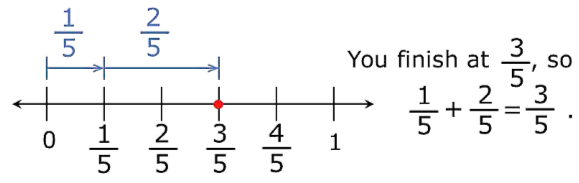ii. If we have to add two rational numbers whose denominators are same then we simply add their numerators and the denominator remains the same.

Example

Solution

As the denominator is the same, we can simply add their numerator.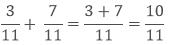b. Addition of two Rational Numbers with different denominator

If we have to add two rational numbers with different denominators then we have to take the LCM of denominators and find their equivalent rational numbers with the LCM as the denominator, and then add them.

Example

Solution

To add the two rational numbers, first, we need to take the LCM of denominators the find the equivalent rational numbers.

LCM of 5 and 7 is 35.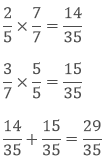Like integers, the additive inverse of rational numbers is also the same.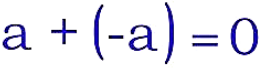This shows that the additive inverse of 3/7 is - (3/7)
This shows that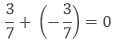2. Subtraction

If we have to subtract two rational numbers then we have to add the additive inverse of the rational number that is being subtracted to the other rational number.

a - b = a + (-b)

Example

Subtract 4/21 from 8/21.

Solution

i. In the first method, we will simply subtract the numerator and the denominator remains the same.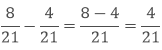ii. In the second method, we will add the additive inverse of the second number to the first number.3. Multiplication

a. Multiplication of a Rational Number with a Positive Integer.

To multiply a rational number with a positive integer we simply multiply the integer with the numerator and the denominator remains the same.

Example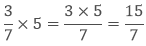b. Multiply of a Rational Number with a Negative Integer

To multiply a rational number with a negative integer we simply multiply the integer with the numerator and the denominator remains the same and the resultant rational number will be a negative rational number.

Example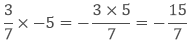c. Multiply of a Rational Number with another Rational Number

To multiply a rational number with another rational number we have to multiply the numerator of two rational numbers and multiply the denominator of the two rational numbers.Example

Multiply 3/7 and 5/11.

Solution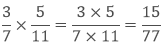4. Division

a. Reciprocal

Reciprocal is the multiplier of the given rational number which gives the product of 1.

Reciprocal of a/b is b/a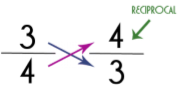Product of Reciprocal

If we multiply the reciprocal of the rational number with that rational number then the product will always be 1.

Example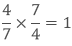b. Division of a Rational Number with another Rational Number

To divide a rational number with another rational number we have to multiply the reciprocal of the rational number with the other rational number.

Example

DivideSolution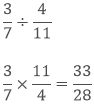```### Course Features

• 728 Video Lectures
• Revision Notes
• Previous Year Papers
• Mind Map
• Study Planner
• NCERT Solutions
• Discussion Forum
• Test paper with Video Solution